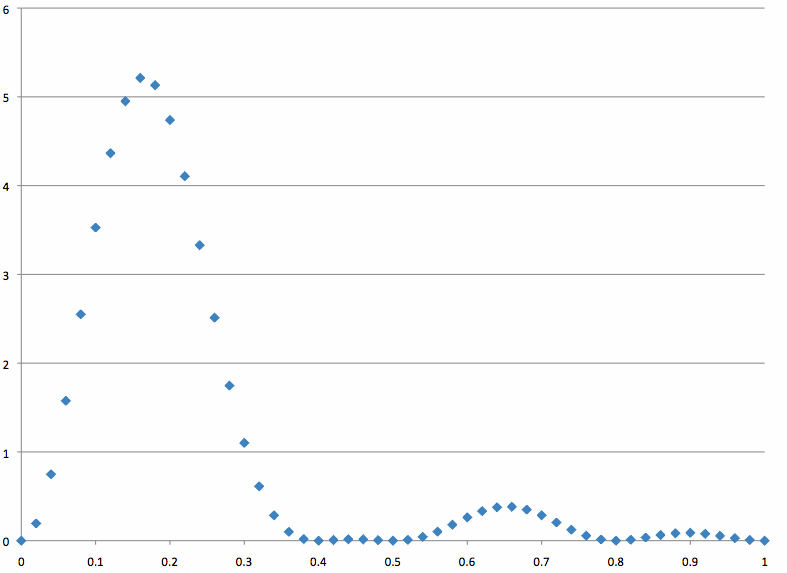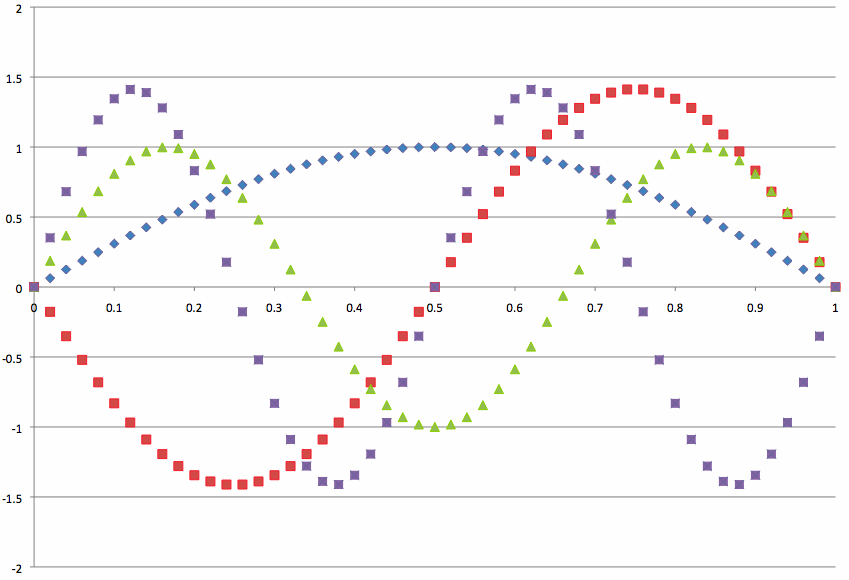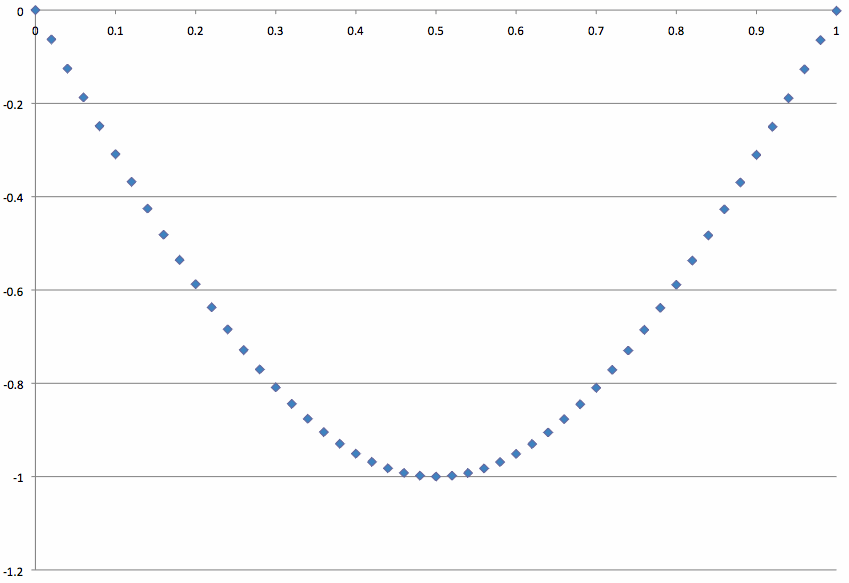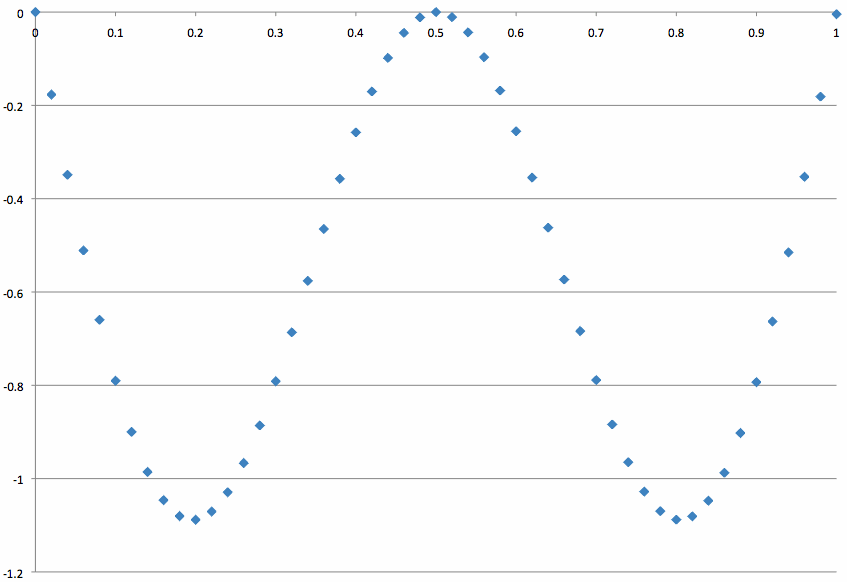# Time-dependence in Quantum Mechanics

Postulate V states how the time-dependent Schrödinger equation describes the evolution of a system’s wavefunction through time.

## Introduction

The Schrödinger equation is the basis for quantum mechanics. The solutions to the equation, known as wave functions, give complete quantum mechanical insight into the system under observation. The Hamiltonian operator, which is specific to the system's environment, acts upon the wavefunction to yield the wavefunction again, accompanied by the energy of the system (the eigenvalue). Postulate V states that the time-dependant Schrödinger equation (1) describes the evolution of a system’s wavefunction through time.

$\hat{H}\Psi_(x,t) = {i}\hbar \dfrac{\partial\Psi}{\partial{t}} \tag{1}$

For a time-independent Hamiltonian Operator, this assumption allows separation of variables:

$\Psi _{(x,t)} =\psi _{(x)} \cdot f_{(t)} \tag{2}$

Equation (2) is substituted into equation (1).

$\hat{H}\psi _{(x)}\cdot f_{(t)} = i\hbar \displaystyle\frac{d\psi _{(x)} \cdot f_{(t)}}{dt} \tag{3}$

Equation (3) is then manipulated so the left side is explicitly in terms of $$x$$, while the right side only in terms of $$t$$.

$\displaystyle\frac{1}{\psi _{(x)}} {\hat{H}_{(x)}} \psi _{(x)} =\displaystyle\frac{1}{f_{(t)} } i\hbar \displaystyle\frac{df_{(t)} }{dt} \tag{4}$

If both sides (dependent upon different variables) are to be equal, they must both equal the same constant. We shall call this constant E. The left side becomes equivalent to the time-independent Schrödinger equation:

${\hat{H}} \Psi _{(x)} =E\Psi _{(x)} \tag{5}$

The right side becomes:

${\displaystyle\frac{df_{(t)}}{dt}} =\displaystyle\dfrac{-i}{\hbar } E\cdot f_{(t)} \tag{6}$

Equation (6) is manipulated into equation (7) and then integrated:

$${\displaystyle\frac{df_{(t)}}{f_{(t)}}} =\displaystyle\dfrac{-i}{\hbar } E\cdot {dt}$$

$\displaystyle\int \frac{df_{(t)} }{f_{(t)} } =\frac{-i}{\hbar } E\cdot \int {dt} \tag{7}$

$f_{(t)} =\displaystyle{e^{(-\frac{iE\cdot {t}}{\hbar })}} \tag{8}$

Since $$E=hv=\hbar\omega$$, this is also equal to:

$f_{(t)} =e^{(-i \cdot {\omega} \cdot {t})} \tag{9}$

Equations (9) now plugged into equation (2) give the solution to the time-dependant Schrödinger equation:

$\Psi _{(x,t)} =\psi _{(x)} \cdot e^{(-i\cdot {\omega}\cdot {t})} \tag{10}$

An example of the time dependence of a localized particle in a box can be seen below. First, the constants have to be defined:

• a=1
• h=1
• m=1
• $$i=\sqrt{-1}$$

Using these constants, the wavefunction and energy solutions can be found:

$E(n)=\dfrac{h^2}\cdot{n^2}} {8\cdot{m}\cdot{a^2}}$

$$\omega{(n)}=\dfrac{2 \pi E_n}{h}$$

\psi{(n,x,t)}=\sqrt{\dfrac{2}{a}}{sin\left(\dfrac{n \pi x}{a}\right)}\cdot{e^{-i \omega{(n)}t}}

The particle can then be localized by superpositioning the wavefunctions:

$$n_{max}=4$$ $$n=1,2...n_{max}$$

$$\Psi{(x,t)}=\displaystyle\sum_{n}\dfrac{1}{\sqrt{n_{max}}}\cdot\psi{(n,x,t)}$$

$$P(x,t)=\Psi{(x,t)}\cdot{\Psi{(x,t)}}$$

Using these values and definitions, the following plots of wavefunctions, superposed wavefunctions, and probability distributions can be found (attached below). These plots are captioned to show whether they are real or imaginary and the time.

• For t=0, there is no imaginary part for the wavefunction, superpsed wavefunctions, and therefore, the probability distribution function.
• For all probability distribution functions, there is no imaginary portion since i2= -1.Real portion of wavefunctions at t=0Real portion of superposed wavefunctions at t=0Real portion of probability distribution at t=0Real portion of wavefunctions at t=1Real portion of superposed wavefunctions at t=1Real portion of probability distribution at t=1Imaginary portion of wavefunctions at t=1Imaginary portion of superposed wavefunctions at t=1Real portion of wavefunctions at t=2Real portion of superposed wavefunctions at t=2Real portion of probability distribution at t=2Imaginary portion of wavefunctions at t=2Imaginary portion of superposed wavefunctions at t=2